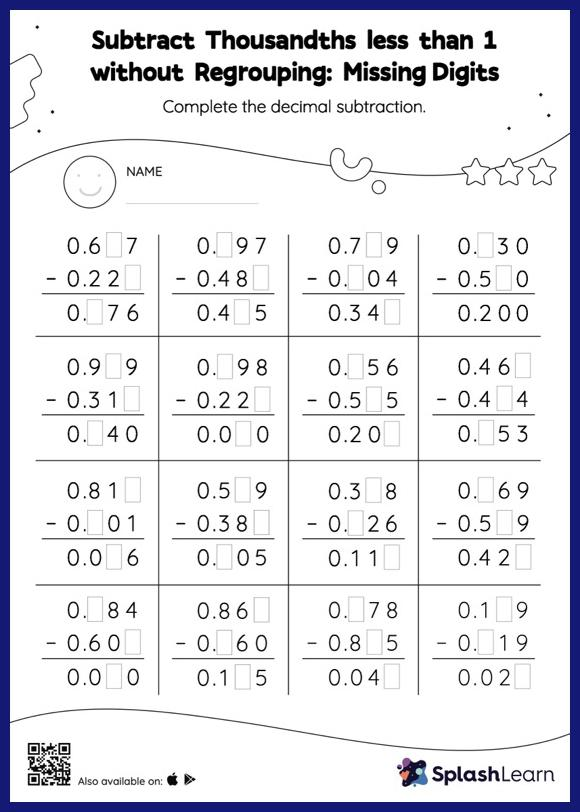# Subtract Thousandths less than 1 without Regrouping: Missing Digits Worksheet

Home > Subtract Thousandths less than 1 without Regrouping: Missing DigitsChildren use their math skills to complete this subtract thousandths less than 1 without regrouping worksheet. Students find the missing number while subtracting decimals by using the relationship between addition and subtraction. Subtract thousandths less than 1 without regrouping worksheet does not require them to regroup numbers.